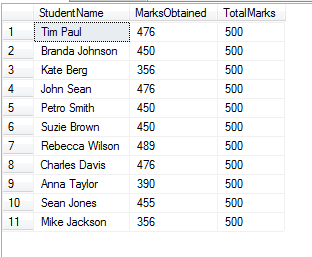# Generate Sequence Numbers in SQL Select Query

This article explains how you can generate sequence numbers in SQL select query. It uses SQL functions Row_Number, Rank, and Dense_rank

The Rank function can be used to generate a sequential number for each row or to give a rank based on specific criteria. The ranking function returns a ranking value for each row. However, based on criteria more than one row can get the same rank.

There are 3 types of functions that can be used to rank the records.

1. Row_number
2. Rank
3. Dense_rank

All these functions make use of the OVER clause. The OVER clause determines the sequence in which rows are assigned a rank, it may be ascending or descending.

To properly view the functionality of these Ranking functions first we will create a table named StudentTotalMarks. To create this table use the below given query in SQL Server.

```    CREATE TABLE StudentTotalMarks
(
StudentName VARCHAR(80),
MarksObtained INT,
TotalMarks INT
)
```

Now we will insert the data into this table once created, with the insert command.

```
INSERT INTO StudentTotalMarks VALUES ('Tim Paul', 476, 500)

INSERT INTO StudentTotalMarks VALUES ('Branda Johnson', 450, 500)

INSERT INTO StudentTotalMarks VALUES ('Kate Berg', 356, 500)

INSERT INTO StudentTotalMarks VALUES ('John Sean', 476, 500)

INSERT INTO StudentTotalMarks VALUES ('Petro Smith', 450, 500)

INSERT INTO StudentTotalMarks VALUES ('Suzie Brown', 450, 500)

INSERT INTO StudentTotalMarks VALUES ('Rebecca Wilson', 489, 500)

INSERT INTO StudentTotalMarks VALUES ('Charles Davis', 476, 500)

INSERT INTO StudentTotalMarks VALUES ('Anna Taylor', 390, 500)

INSERT INTO StudentTotalMarks VALUES ('Sean Jones', 455, 500)

INSERT INTO StudentTotalMarks VALUES ('Mike Jackson', 356, 500)

```

These are the marks of 11 students, which are shown in the table below.Now our aim is to Rank all the students on the basis of their MarksObtained. We can do this by Row_Number, Rank or Dense_Rank functions. Let me show you the working of all 3 of these functions.

1. ## Row_Number

This function will rank the column sequentially. Starting with 1 and then incrementing by 1 (eg.1,2,3,4), regardless of the same data in the column to be ranked.

The following query displays the sequential number on a column by using the Row_Number function.

```    SELECT StudentName, MarksObtained,
ROW_NUMBER() OVER(ORDER BY MarksObtained DESC) AS Rank
FROM StudentTotalMarks
```2. ## Rank

This function will rank the column based on the specified criteria. If the table two students have the same marks then this function will give the same rank, however, the next rank number will be skipped (eg.1,2,3,3,5,6).

The following query displays the working of Rank function.

```    SELECT StudentName, MarksObtained,
RANK() Over(ORDER BY MarksObtained DESC) AS Rank
FROM StudentTotalMarks
```Here three students got the same rank 2, so the next rank is 5 omitting 3 and 4 rank numbers.

3. ## Dense_Rank

The Dense_rank() function is used where consecutive ranking values need to be given based on specific criteria. It Ranks the column in the same way as of Rank() function however rank is not skipped here (eg. 1,2,3,4,5,5,6)

To view the functioning of the Dense_rank, write down the following query.

SELECT StudentName, MarksObtained, DENSE_RANK() OVER(ORDER BY MarksObtained DESC) AS Rank FROM StudentTotalMarksHere three students got same rank 2, so next rank is 3. here it is not 5 as it was in Rank() function.

You can also use those functions with Inner Join or Outer Join OR Cross Apply.

Below code uses Rank with Join

```    SELECT StudentName, MarksObtained, c.CourseID, c.CourseName
RANK() Over(ORDER BY MarksObtained DESC) AS Rank
FROM StudentTotalMarks S
JOIN CourseDetails C ON C.CourseID = S.CourseID
```